2
2
0 0
1 1
2
0 1
1 0
2
2
0 0
1 1
2
1 0
2 1
2
3
0 0
1 1
2 1
2
2 0
3 0

Yes
No
No

# 分析：

###### 那如何来判断两线段是否相交呢？

1.线段AB与CD所在的直线相交，即点A和点B分别在直线CD的两边；
2.线段CD与AB所在的直线相交，即点C和点D分别在直线AB的两边；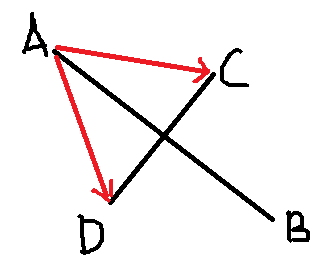1.只有1点相交，如下图：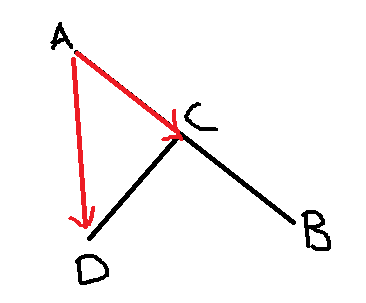2.两条线段重合，如下图：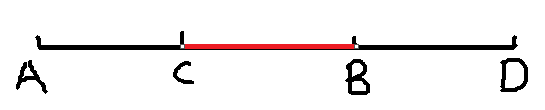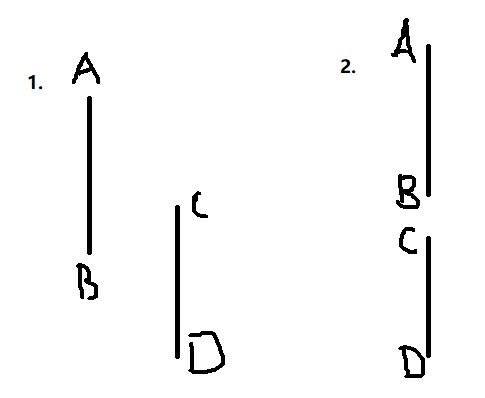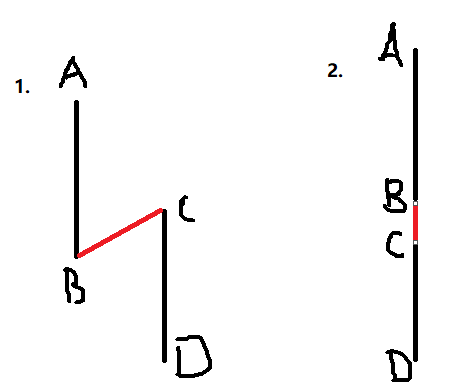###### 若一条线段AB与另一条线段CD共线，且线段AB的起点小于等于线段CD的起点，但线段AB的终点（注意是终点）大于等于线段CD的起点（注意是起点），或者交换一下顺序，CD的起点小于AB的起点…只要满足其中一个，就表示有重合部分。

y1)为第一条线段的起点，p2(x2, y2)为第一条线段的终点，p3(x3, y3)为第二条线段的起点，p4(x4,
y4)为第二段线段的终点，由此可构造两个向量：

v1(x2-x1, y2-y1)，v2(x4-x3, y4-y3)

p1), s2(p3,
p2)，如果s1×v2与s2×v2异号(s1->v2与s2->v2转动的方向相反），则说明p1和p2位于L2的两侧。同理可判定p3和p4是否跨立L1。如果上述四个叉积中任何一个等于0，则说明一条线段的端点在另一条线上。

x0-x1=k1(x2-x1) y0-y1=k1(y2-y1) x0-x3=k2(x4-x3) y0-y3=k2(y4-y3)

x0(y2-y1)-x1(y2-y1)=y0(x2-x1)-y1(x2-x1)
x0(y4-y3)-x3(y4-y3)=y0(x4-x3)-y3(x4-x3) 将含有未知数x0和y0的项移到左边，常数项移动到右边，得：

(y2-y1)x0+(x1-x2)y0=(y2-y1)x1+(x1-x2)y1
(y4-y3)x0+(x3-x4)y0=(y4-y3)x3+(x3-x4)y3 设两个常数项分别为b1和b2：

b1=(y2-y1)x1+(x1-x2)y1 b2=(y4-y3)x3+(x3-x4)y3

|D|=(x2-x1)(y4-y3)-(x4-x3)(y2-y1) |D1|=b2(x2-x1)-b1(x4-x3)
|D2|=b2(y2-y1)-b1(y4-y3) 由此，可求得交点坐标为：

x0=|D1|/|D|, y0=|D2|/|D| 解毕。

# AC代码：

``````#include<stdio.h>
#include<string.h>
#include<algorithm>
using namespace std;
int n,flag;
int k;
struct node
{
int x,y;
} s;
double f(node a,node b,node c)///如果AB.AC，得到的结果是垂直于ABC这个平面的一个向量
{///两个向量的向量积，是一个垂直于两个向量的向量
return (b.x-a.x)*(c.y-a.y)-(b.y-a.y)*(c.x-a.x);///AB.AC=（b.x-a.x）*(c.y-a.y)-(b.y-a.y)*(c.x-a.x)；
}
int dfs(node a,node b,node c,node d)
{
if(max(a.x,b.x)>=min(c.x,d.x)&&max(c.x,d.x)>=min(a.x,b.x)&&
max(a.y,b.y)>=min(c.y,d.y)&&max(c.y,d.y)>=min(a.y,b.y)&&

return 0;
}
int main()
{
while(~scanf("%d",&n))
{
memset(k,0,sizeof(k));
flag=0;
for(int i=0; i<n; i++)
{
scanf("%d",&k[i]);
for(int j=0; j<k[i]; j++)
scanf("%d%d",&s[i][j].x,&s[i][j].y);
}
if(n==1)
{
printf("No\n");
continue;
}
for(int i=0; i<n-1; i++)///枚举
for(int j=1; j<k[i]; j++)
for(int u=i+1; u<n; u++)
for(int v=1; v<k[u]; v++)
if(dfs(s[i][j-1],s[i][j],s[u][v-1],s[u][v]))
{
flag=1;
break;
}
if(flag)
printf("Yes\n");
else
printf("No\n");
}
return 0;
}
``````